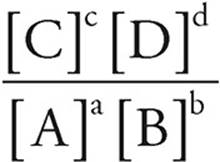﻿ ﻿THE EQUILIBRIUM CONSTANT, Keq - Equilibrium, Acids and Bases, Titrations, and Solubility - Content Review for the AP Chemistry Exam - Cracking the AP Chemistry Exam

## Cracking the AP Chemistry Exam

Part IV

Content Review for the AP Chemistry Exam

Chapter 8

Big Idea #6: Equilibrium, Acids and Bases, Titrations, and Solubility

Any bond or intermolecular attraction that can be formed can be broken. These two processes are in dynamic competition, sensitive to initial conditions and external perturbations.

THE EQUILIBRIUM CONSTANT, Keq

Most chemical processes are reversible. That is, reactants react to form products, but those products can also react to form reactants. A reaction is at equilibrium when the rate of the forward reaction is equal to the rate of the reverse reaction. The relationship between the concentrations of reactants and products in a reaction at equilibrium is given by the equilibrium expression, also called the law of mass action.

The Equilibrium Expression

For the reaction

aA+bBcC+dD

Keq=1. [A], [B], [C], and [D] are molar concentrations or partial pressures at equilibrium.

2. Products are in the numerator, and reactants are in the denominator.

3. Coefficients in the balanced equation become exponents in the equilibrium expression.

4. Solids and pure liquids are not included in the equilibrium expression because they cannot change their concentration. Only gaseous and aqueous species are included in the expression.

5. Units are not given for Keq.

Let’s look at a few examples:

1. HC2H3O2(aq)H+(aq)+C2H3O2(aq)

Keq=Ka=This reaction shows the dissociation of acetic acid in water. All of the reactants and products are aqueous particles, so they are all included in the equilibrium expression. None of the reactants or products have coefficients, so there are no exponents in the equilibrium expression. This is the standard form of Ka, the acid dissociation constant.

2. 2H2S(g)+3O2(g)2H2O(g)+2SO2(g)

Keq=Kc=Keq=Kp=All of the reactants and products in this reaction are gases, so Keq can be expressed in terms of concentration (Kc, moles/liter or molarity) or in terms of partial pressure (Kp, atmospheres). All of the reactants and products are included here, and the coefficients in the reaction become exponents in the equilibrium expression.

3. CaF2(s)Ca2+(aq)+2F(aq)

Keq=Ksp=[Ca2+][F]2

This reaction shows the dissociation of a slightly soluble salt. There is no denominator in this equilibrium expression because the reactant is a solid. Solids are left out of the equilibrium expression because the concentration of a solid is constant. There must be some solid present for equilibrium to exist, but you do not need to include it in your calculations. This form of Keq is called the solubility product, Ksp.

4. NH3(aq)+H2O(l)NH4+(aq)+OH(aq)

Keq = Kb =This is the acid-base reaction between ammonia and water. We can leave water out of the equilibrium expression because it is a pure liquid. This is the standard form for Kb, the base dissociation constant.

Here is a roundup of the equilibrium constants you need to be familiar with for the test.

· Kc is the constant for molar concentrations.

· Kp is the constant for partial pressures.

· Ksp is the solubility product, which has no denominator because the reactants are solids.

· Ka is the acid dissociation constant for weak acids.

· Kb is the base dissociation constant for weak bases.

· Kw describes the ionization of water (Kw=1 × 10−14).

A large value for Keq means that products are favored over reactants at equilibrium, while a small value for Keq means that reactants are favored over products at equilibrium.

The equilibrium constant has a lot of aliases, but they all take the same form and tell you the same thing. The equilibrium constant tells you the relative amounts of products and reactants at equilibrium.

﻿